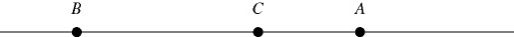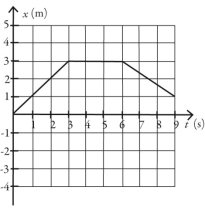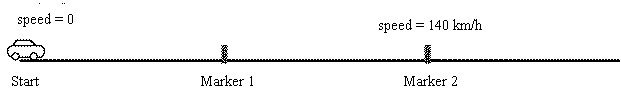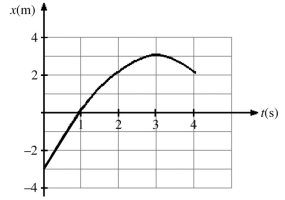/
/
/
2.2   Problems 1) A cat runs along a straight line (the
Not my Question
Flag Content

# Question : 2.2   Problems 1) A cat runs along a straight line (the : 2005792

2.2   Problems

1) A cat runs along a straight line (the x-axis) from point A to point B to point C, as shown in the figure. The distance between points A and C is 5.00 m, the distance between points B and C is 10.0 m, and the positive direction of the x-axis points to the right. The time to run from A to B is 20.0 s, and the time from B to C is 8.00 s.  As the cat runs along the x-axis between points A and C

(a) what is the magnitude of its average velocity?

(b) what is its average speed?2) The figure shows the position of an object as a function of time.  During the time interval from time t = 0.0 s and time t = 9.0 s

(a) what is the length of the path the object followed?

(b) what is the displacement of the object?3) As part of an exercise program, a woman walks south at a speed of 2.00 m/s for 60.0 minutes.  She then turns around and walks north a distance 3000 m in 25.0 minutes

(a) What is the woman's average velocity during her entire motion?

A) 0.824 m/s south

B) 1.93 m/s south

C) 2.00 m/s south

D) 1.79 m/s south

E) 800 m/s south

(b) What is the woman's average speed during her entire motion?

A) 0.824 m/s

B) 1.93 m/s

C) 2.00 m/s

D) 1.79 m/s

E) 800 m/s

4) The figure shows the position of an object as a function of time, with all numbers accurate to two significant figures.  Between time t = 0.0 s and time t = 9.0 s

(a) what is the average speed of the object?

(b) what is the average velocity of the object?5) If the fastest you can safely drive is 65 mi/h what is the longest time you can stop for dinner if you must travel 541 mi in 9.6 h total?

A) 1.0 h

B) 1.3 h

C) 1.4 h

D) You can't stop at all.

6) Arthur and Betty start walking toward each other when they are 100 m apart. Arthur has a speed of 3.0 m/s and Betty has a speed of 2.0 m/s. Their dog, Spot, starts by Arthur's side at the same time and runs back and forth between them at 5.0 m/s. By the time Arthur and Betty meet, what distance has Spot run?

7) A racing car accelerates uniformly from rest along a straight track.  This track has markers spaced at equal distances along it from the start, as shown in the figure.  The car reaches a speed of 140 km/h as it passes marker 2. Where on the track was the car when it was traveling at 70 km/h?A) Before marker 1

B) At marker 1

C) Between marker 1 and marker 2

8) The figure represents the position of a particle as it travels along the x-axis.  Between t = 2 s and t = 4 s, what is (a) the average speed of the particle and (b) the average velocity of the particle?9) The position of an object as a function of time is given by x = bt2 - ct, where b = 2.0 m/s2 and c = 6.7 m/s and x and t are in SI units. What is the instantaneous velocity of the object when

t = 2.2?

A) 1.7 m/s

B) 2.1 m/s

C) 2.3 m/s

D) 2.7 m/s

10) The position of an object is given by x = at3 – bt2+ct, where a = 4.1 m/s3,

b = 2.2 m/s2, c = 1.7 m/s, and x and t are in SI units. What is the instantaneous acceleration of the object when t = 0.7s?

A) -13 m/s2

B) 2.9 m/s2

C) 4.6 m/s2

D) 13 m/s2

## Solution 5 (1 Ratings )

Solved
Physics 1 Week Ago 119 Views